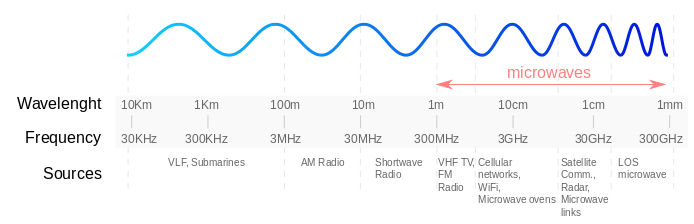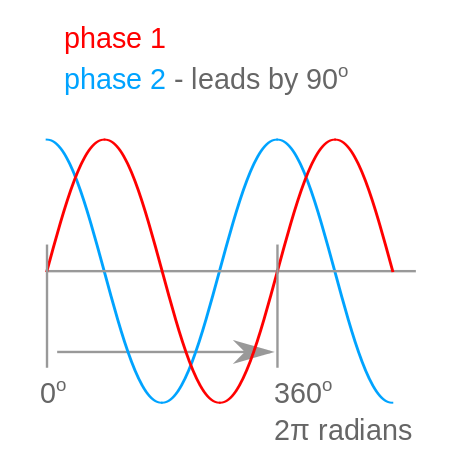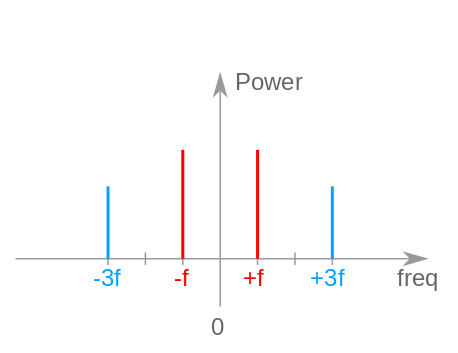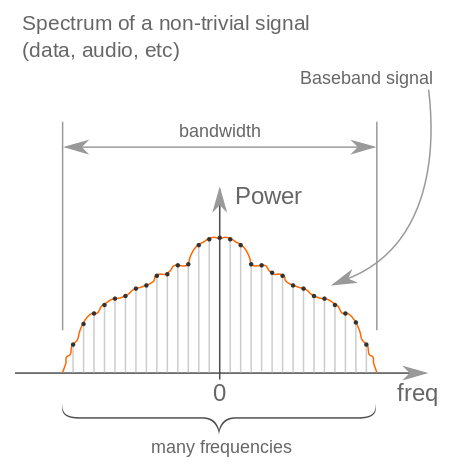Contents

The wave, as an element in physics, is an oscillation transferring energy or information, which travels through space and matter. The most common examples of waves are: light, sound or water waves.

There are two main types of radio waves: mechanical waves and electromagnetic waves. While mechanical waves transmit energy in a material medium, electromagnetic waves travel though space itself.

Electromagnetic waves consist of periodic oscillations of electrical and magnetic fields generated by charged particles. These types of waves vary in wavelength and include radio waves, microwaves, infrared radiation, visible light, ultraviolet radiation, X-rays, and gamma rays. Its respective frequency range is what determines a wave’s type.

Radio waves are a type of electromagnetic radiation with frequencies ranging from 300 GHz to as low as 3 Hz, and wavelengths ranging from 1 millimeter (0.039 inches) to 100 kilometers (62 miles).

Similar to all other electromagnetic waves, they travel at the speed of light. Naturally occurring radio waves are made by lightning or by astronomical objects. Artificially generated radio waves are used in a large number of applications: such as fixed and mobile radio communication, broadcasting, radar, communications satellites, computer networks etc.

Radio waves frequencies for 2.5G networks are further explained in the GSM Concepts section.

## Phase, Amplitude, Wavelength and Frequency

In typical representations, the wave has a sinusoidal movement. Below you can see a diagram illustrating the phase and the amplitude of a wave in the time domain.

File:wave.png

The phase is the distance between the point of origin of any given wave and its first zero crossing.

Phase can also refer to the difference between two waves which are at the same frequency and referenced to the same point in time. If the two waves have no difference, they are in phase. However, if they they have the same frequency and different phases, they are out of phase with each other. This phase difference can be expressed from 0º to 360º (degrees), or from 0 to 2π (radians).

The amplitude (or height) is the distance between the middle line of a wave and either its crest or through.

Any give wave will have a wavelength which is defined by the distance between two consecutive locations, such as crests or zero crossings, that are in phase with each other.

The frequency is the number of oscillations per unit time.

The wavelength is inversely proportional to the frequency, i.e. the higher the frequency of a wave, the shorter its wavelength and viceversa.

Radio frequencies range from around 3kHz to 300 GHz. See below an illustration of high and low radio frequencies.Radio spectrum is the section of electromagnetic spectrum that comprises radio waves with frequencies ranging from 3 kHz to 300 GHz. Essentially, there are two main domains of representing radio waves: the time domain and the frequency domain.

A time domain illustration of a particular signal shows the how it changes over time. As seen in the diagram below, there are two waves in the same frequency, represented in the time domain. However, the second wave (in blue) leads by 90º, thus causing the two waves to be out of phase with each other.In the next diagram, you can see two waves with different frequencies and, in purple, a third, which is the sum of the first two. The frequency of a wave is correlated to its number of oscillations: the higher the frequency, the more oscillations the wave will have.The frequency domain representation typically shows how much signal each frequency band (from a range of frequencies) carries. A spectrum of more frequencies is the frequency domain representation of the signal.

The illustration below represents the two waves with different frequencies from the previous diagram, in the frequency domain. The image, therefore, shows the blue wave on the higher frequency scale. The red wave, with a higher amplitude, has more power.To view the signal in the frequency domain, one must use a tool called spectrum analyzer. This device can either analyze the entire signal, or short segment of it. The diagram below illustrates the spectrum of a non-trivial signal (be it data, audio etc.), as seen in the spectrum analyzer.## Simple Radio Modulation and Tuning

In electronics and telecommunications, modulation is the process of mixing the low frequency baseband signal with a modulating (radio frequency) carrier signal for the purpose of transmitting information.

There are various types of analog modulations: amplitude modulation, frequency modulation, phase modulation etc.

In radio communications, the bandwidth is the range of higher and lower frequencies in a continuous set of frequencies. Its main characteristic is that it can hold the same amount of information irrespective of where it is located on the frequency spectrum.

There are various reasons why you need to use modulation:

• to transmit more simultaneous signals over the same channel of communications at different frequencies
• to reduce the baseband signal's wavelength, by shifting it to a higher frequency and, thus, facilitating the transmission with a much smaller antenna

For example, AM or amplitude modulation is a technique that modulates the amplitude of the carrier signal in proportion to the message signal. In AM radio communications, the radio signal is transmitted in a continuous wave with its amplitude modulated by an audio waveform before transmission.

In the example below, you can see the graphical representation of a basic principle of modulation. First, the baseband signal, s(t), is modulated by multiplying it with a radio frequency carrier signal, 2πfct, obtaining a modulated signal, s(t)sin2πfct. Next, the modulated baseband signal, containing the same information, is shifted on a much higher frequency. Another important aspect is the presence of the negative frequency, a mirror image of the spectrum on the positive side.

Note: When multiplying real valued signals, the positive and the negative frequencies are mirrors of the same spectrum. For this reason, we only analyze and take into account the spectrum on the positive side.### Fundamental digital modulation methods

In digital modulation, a discrete signal modulates an analog carrier signal. Digital modulation methods can be considered as digital-to-analog conversion, and the corresponding demodulation or detection as analog-to-digital conversion.

Below are the primary digital modulation techniques:

• PSK (phase-shift keying): using a finite number of phases
• FSK (frequency-shift keying): using a finite number of frequencies
• ASK (amplitude-shift keying): using a finite number of amplitudes
• QAM (quadrature amplitude modulation): using a finite number of at least two phases and at least two amplitudes

Note: GSM uses GMSK (Guassian minimum-shift keying), a form of FSK.

## Legal Aspects

Radio spectrum is a public national resource and almost all national government have strict legislation that allows them to regulate it.

### Spectrum Management

At a national level there are coordinating organizations that perform spectrum management through a series of measures:

• plan, allocate and designate the entire nation radio spectrum
• monitor and control the use of the national radio spectrum

The RF spectrum is a reusable national resource and is a property of each national state. Is a natural monopoly and, as such, there is typically one regulator per RF band.

The International Telecommunication Union (ITU) is the international coordinating organization managing the shared use of radio spectrum.

### ITU Regions

ITU has defined three regions for managing the global radio spectrum. These regions are:

• 1. Europe, Africa, the Middle East west of the Persian Gulf including Iraq, the former Soviet Union and Mongolia
• 2. the Americas, Greenland and a few Eastern Pacific Islands
• 3. most of non-former-Soviet-Union Asia, east of and including Iran, and most of Oceania

Please refer to this link for a world map of all the regions.

### GSM Standardized Bands

There are four globally standardized GSM bands, as you can see in the table below:

GSM 850850824 – 849869 – 894North America, the Caribbean and Latin America
E-GSM 900900880 – 915925 – 960Europe, the Middle East, Africa and Asia-Pacific
GSM 180018001,710 – 1 ,7851,805 – 1,880Europe, the Middle East, Africa and Asia-Pacific
GSM 190019001,850 – 1,9091,930 – 1,989North America, the Caribbean and Latin America

Note: E-GSM or Extended GSM-900 Band and includes the Standard GSM-900 band.

1.  Wikipedia contributors. “Electromagnetic radiation”. Wikipedia, The Free Encyclopedia; 2014 Mar 12, 12:17 UTC [cited 2014 Mar 20]. Available from: http://en.wikipedia.org/wiki/Electromagnetic_wave
2. Wikipedia contributors. “Radio wave”. Wikipedia, The Free Encyclopedia; 2014 Feb 24, 14:41 UTC [cited 2014 Mar 20]. Available from: http://en.wikipedia.org/wiki/Radio_wave
3. Wikipedia contributors. “Phase (waves)”. Wikipedia, The Free Encyclopedia; 2014 Feb 21, 05:20 UTC [cited 2014 Mar 20]. Available from: http://en.wikipedia.org/wiki/Phase_(waves)
4. Wikipedia contributors. “Amplitude”. Wikipedia, The Free Encyclopedia; 2014 Feb 21, 04:40 UTC [cited 2014 Mar 20]. Available from: http://en.wikipedia.org/wiki/Amplitude
5. Wikipedia contributors. “Wavelength”. Wikipedia, The Free Encyclopedia; 2015 Jan 20, 16:42 UTC [cited 2015 Jan 21]. Available from: http://en.wikipedia.org/wiki/Wavelength
6. Wikipedia contributors. “Radio Frequency”. Wikipedia, The Free Encyclopedia; 2014 Mar 17, 17:18 UTC [cited 2014 Mar 20]. Available from: http://en.wikipedia.org/wiki/Radio_frequency
7. Wikipedia contributors. “Radio Spectrum”. Wikipedia, The Free Encyclopedia; 2014 Mar 04, 20:16 UTC [cited 2014 Mar 20]. Available from: http://en.wikipedia.org/wiki/Radio_spectrum
8. Wikipedia contributors. “Modulation”. Wikipedia, The Free Encyclopedia; 2014 Mar 19, 08:37 UTC [cited 2014 Mar 20]. Available from: http://en.wikipedia.org/wiki/Modulation
9. Wikipedia contributors. “Bandwidth”. Wikipedia, The Free Encyclopedia; 2015 Jan 09, 16:15 UTC [cited 2015 Jan 20]. Available from: http://en.wikipedia.org/wiki/Bandwidth_%28signal_processing%29
10. Wikipedia contributors. “Amplitude modulation”. Wikipedia, The Free Encyclopedia; 2015 Jan 22, 14:13 UTC [cited 2015 Jan 22]. Available from: http://en.wikipedia.org/wiki/Amplitude_modulation